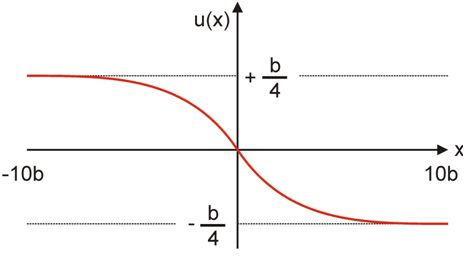# 21.2: Making a Dislocation

$$\newcommand{\vecs}{\overset { \rightharpoonup} {\mathbf{#1}} }$$ $$\newcommand{\vecd}{\overset{-\!-\!\rightharpoonup}{\vphantom{a}\smash {#1}}}$$$$\newcommand{\id}{\mathrm{id}}$$ $$\newcommand{\Span}{\mathrm{span}}$$ $$\newcommand{\kernel}{\mathrm{null}\,}$$ $$\newcommand{\range}{\mathrm{range}\,}$$ $$\newcommand{\RealPart}{\mathrm{Re}}$$ $$\newcommand{\ImaginaryPart}{\mathrm{Im}}$$ $$\newcommand{\Argument}{\mathrm{Arg}}$$ $$\newcommand{\norm}{\| #1 \|}$$ $$\newcommand{\inner}{\langle #1, #2 \rangle}$$ $$\newcommand{\Span}{\mathrm{span}}$$ $$\newcommand{\id}{\mathrm{id}}$$ $$\newcommand{\Span}{\mathrm{span}}$$ $$\newcommand{\kernel}{\mathrm{null}\,}$$ $$\newcommand{\range}{\mathrm{range}\,}$$ $$\newcommand{\RealPart}{\mathrm{Re}}$$ $$\newcommand{\ImaginaryPart}{\mathrm{Im}}$$ $$\newcommand{\Argument}{\mathrm{Arg}}$$ $$\newcommand{\norm}{\| #1 \|}$$ $$\newcommand{\inner}{\langle #1, #2 \rangle}$$ $$\newcommand{\Span}{\mathrm{span}}$$$$\newcommand{\AA}{\unicode[.8,0]{x212B}}$$

We will consider the changes in atomic positions in a given plane of atoms when an edge dislocation is formed in a simple cubic lattice. If the extension of the dislocation is large compared with the atomic spacing b, the horizontal displacement u varies slowly from atom to atom. The relative displacement of neighbouring atoms within each half crystal is much smaller than b.

We start out by considering the initial atomic positions in just two planes A and B.The variation of the displacement u with distance from the dislocation line

It is clear from the above figure that a tan function would be consistent with the displacement. Peierls showed this was the case.

• The following animation shows how the model is set up using the initial atomic positions in just two planes; here we are making the approximation that the displacements of atoms in the planes on either side (in the y direction) are small enough to be neglected from the overall energy. We are able to show that the final displacement (in the x direction) varies as an arctan function, as demonstrated by Peierls.

This page titled 21.2: Making a Dislocation is shared under a CC BY-NC-SA license and was authored, remixed, and/or curated by Dissemination of IT for the Promotion of Materials Science (DoITPoMS).